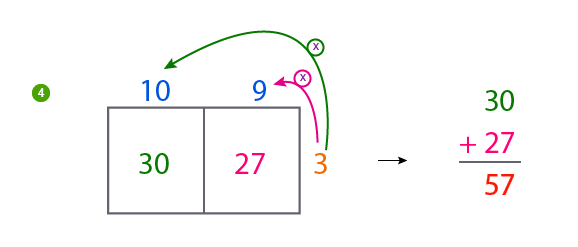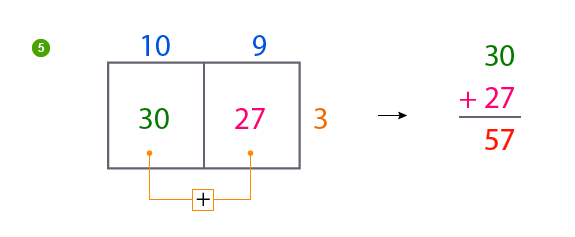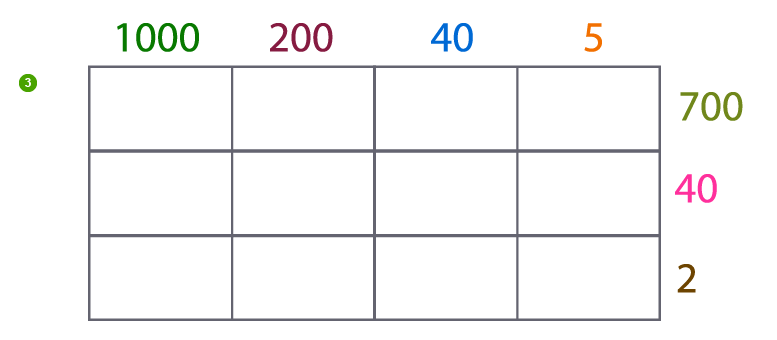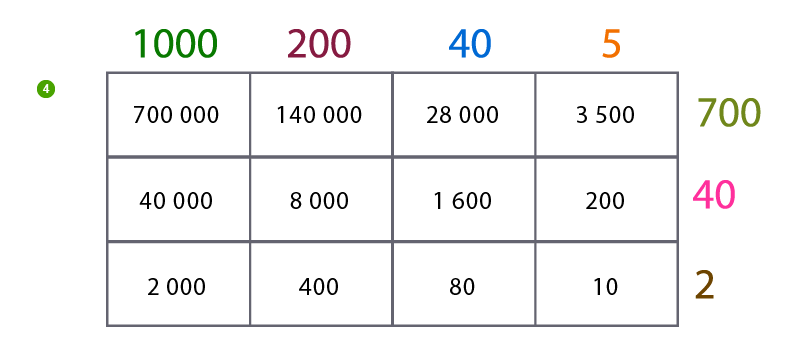# Box method multiplication worksheets PDF - Partial product multiplication worksheets

## Area model multiplication examples and test

Multi-Digit box method multiplication worksheets PDF are giving for students learning or revision. These Partial product multiplication worksheets and Area model multiplication examples and test are gives to make kids more successful in complex multiplication.

Another super easy method to multiply bigger numbers is the box method. We have here very excellent box method multiplication worksheets PDF that will enable a greater understanding of the multi-digit multiplication concept.

Our partial product multiplication worksheets will make kids have a concrete understanding of place value and of course intermediate steps in calculating larger products. The step by step process here gives room for proper corrections of mid-way mistakes.

It is all about fun to use the area model multiplication examples and test. The area model uses beautiful visual tools which helps kids progressively think in a mathematical way, and however become more successful in complex multiplications.

## IMPORTANT FACTS ABOUT BOX MULTIPLICATION METHOD

### Place value - Essential tip for understanding box method

To better understand the Box Method, we must first of all have a basic understanding of place value. In mathematics, place value is the value of each digit in a number, based on its position. Given for instance the number 4852, where is the place value of each digit in that number?

Hey, it's easy to find out. It goes thus;

• 4 is in the "thousandth's" position, so its place value is 4000.
• 8 is in the "hundredths" position, so its place value is 800.
• 5 is in the "ten's" position, so its place value is 50.
• 2 is in the "unit" position, so its place value is 2.

As each factor is broken up into expanded form, children can best understand the place value of each digit.

Hey now, you've got to worry less about multiplying larger digit numbers, for our worksheets consist of well-designed area model multiplication examples and test.

It is very easy.

You simply need to draw a box or an array, with columns and rows proportional to the number of digits or size of numbers to be multiplied. As per the examples, we'll solve below 2 by 1 digits, 3 by 2 digits and 4 by 3 digits.

### Example 1.   2 digit multiplication: Box method | Multiplying 2 digit by 1 digit

Multiply 19 x 3

In the first place, we find the place value of 19 and 3.

19 x 3 = (10 + 9) x 3Secondly, we now draw a box of 2 columns (for 10 and 9) and 1 row (for 3).

Thirdly, write the expanded form at the top (10 and 9) and on one side (3) of the box.Fourthly, it's time to multiply. Hey, we're multiplying only the numbers that meet in each space.Lastly, we add up all the smaller products to make our final product;Hurray! Yes I got it so easily.

So, 19 x 3 = 57

### Example 2.   4 digit multiplication: Box method | Multiplying 4 digit by 3 digit

Multiply 1245 x 742

Hmmm, the multi digits seems difficult right! Not to bother.

Using the box method is very easy and will make you obtain the correct answer with no stress.

As usual, we find the place value of the given factors.

1245 x 742 = (1000 + 200 + 40 + 5) x (700 + 40 + 2)

That means we have a box of 4 colums and 3 rows.

Secondly, we draw our box of 4 columns (for 1000, 200, 40 and 5) and 3 rows (for 700, 40 and 2)

Thirdly, write the expanded form at the top (1000, 200, 40 and 5) and on one side (700, 40, 2) of the box.Next, we place our 4 factors (1000, 200, 40, 5) on each column and by each row, we place our 3 factors (700, 40, 2).After placing the factors, we begin multiplying. Hey, multiply only factors that meet in each space.Ultimately, we now add up all the smaller products to make our final product;

700 000
140 000
28 000
3 500
40 000
8 000
1 600
200
2 000
400
80
+ 10

923 790

Holaaaaa. I got my answer correctly.

Box method is very simple.

•